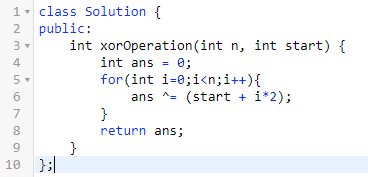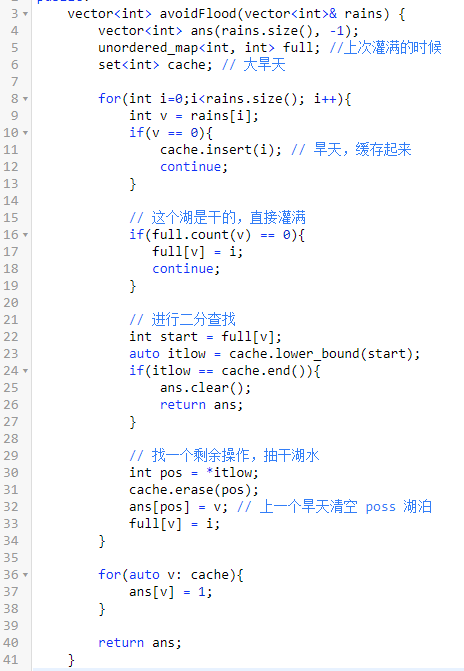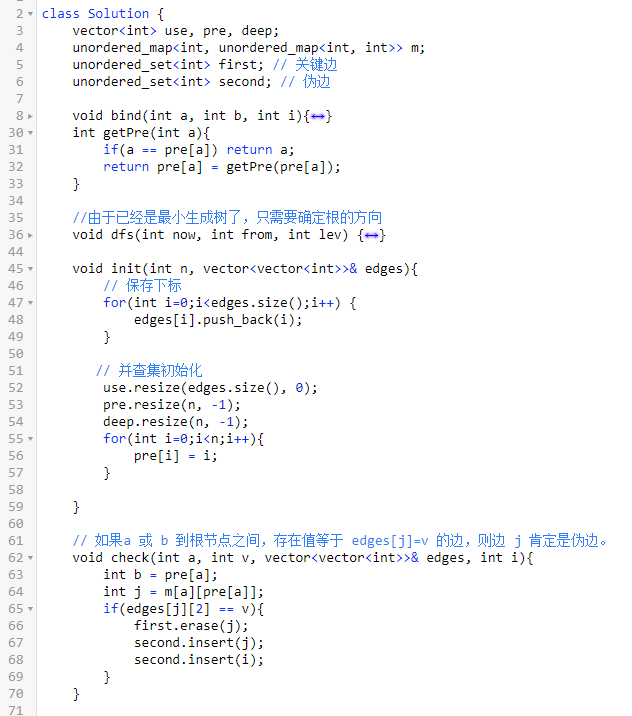# leetcode 第194算法比赛

## 一、数组异或操作1、`f(i, a) = a + 2 * i`
2、`F(i, b) = b + i`
3、`ans(n, a) = ans(n-1) ^ f(n, a)` 4、`Ans(n, b) = Ans(n-1) ^ F(n, b)`

`P(n) = P(n-1) ^ n`
`Ans(n, b) = P(n+b) ^ P(b-1)`

`P(n)`可以分为下面三部分的异或。

1、非高位数字 `P(2^k)`
2、高位数字的地位 `P(j)`
3、高位数字的最高位 j-1 个 `2^k`，判断奇偶性

`P(n) = P(2^k) ^ P(j) ^ ((j&1)<<k)`

1、`n%4=00, P(n) = n`
2、`n%4=01，P(n) = 1`
3、`n%4=10，P(n) = n+1`
4、`n%4=11, P(n) = 0`

## 二、保证文件名唯一``````preName => 0
preName => 1
preName => 2
preName => 5
preName => 7
``````

``````preName => 0
preName => 1,1
preName => 2,2
preName => 5,3
preName => 7,4
``````

## 三、避免洪水泛滥## 四、最小生成树的关键边和伪关键边

1、随便构造一个最小生成树。
2、剩余的边一次尝试加入最小生成树。
3、加上当前加入的是边 v，形成的环上看是否存在与当前边相等权重的边。
4、如果存在，则当前边是伪边，那些相等的边也都是伪边。
5、树上未被证伪的边则是关键边。PS1：四五年没写最小生成树了。

## 五、最后

《完》

-EOF-

QQ算法群：165531769（不止算法）

## 关注公众号,接收最新消息

1.## 关注小密圈,学习各种算法

1.